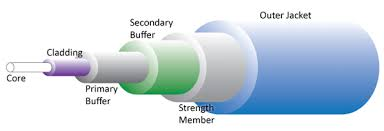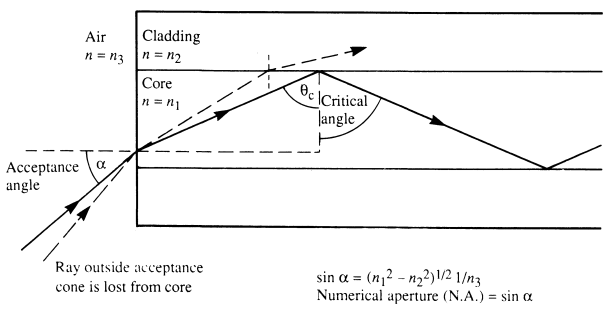Structure Of Optical Fiber-Why Cladding is required?? - technopediasite-Ultimate Resource For Telecom Technical Support

## Friday, May 11, 2018

Posted By: Technopediasite.comAn optical fiber is made of very thin glass rods composed of two parts: the inner portion of the rod or core and the surrounding layer or cladding. Light injected into the core of a glass fiber will follow the physical path of that fiber due to the total internal reflection of the light between the core and the cladding. A plastic sheathing around the fiber provides the mechanical protection.

Principle of the transmission:

* a ray of light enters into the fiber at a small angle a.
* the capability (maximum acceptable value) of the fiber cable to receive light on its core is determined by its numerical aperture NA:

NA= (n12 –n22)1/2
Numerical aperture (NA): NA= (n12 –n22)1/2
•Typical NA values are 0.1 to 0.4 which correspond to acceptance angles of 11 degrees to 46 degrees
•Acceptance angle of a fiber: qa = sin-1 NA
•Light that enters at an angle equal to or less than the acceptance angle will be guided
•NA is more means more light gathering powerLight propagation:

If a > a0: the ray is fully refracted and not captured by the core.

Refraction :
n1 sin Φ1 = n2 sinΦc

If a < a0: the ray is reflected and remains in the core
Reflection :
Φ1 = Φc

Velocity:
The velocity at which light travels through a medium is determined by the refractive index of the medium. The refractive index (n) is a unitless number which represents the ratio of the velocity of light in a vacuum to the velocity of the light in the medium.

n=c/v
where:n: Refractive Index
c: Speed of light in a vacuum (approximately 3 x 10 squre 8 m/s)

V: Speed of light in the transmission medium.
Typical values of n lie between 1.45 and 1.55.

Light entering with different angles does not follow the same path. Light entering the center of the fiber core at a very low angle will take a relatively direct path through the center of the fiber. Light injected at a high angle of incidence or near the outer edge of the fiber core will take a less direct, longer path through the fiber and therefore travel more slowly down the
length of the fiber. Each path resulting from a given angle of incidence and entry point can give rise to a mode. As they travel along the fiber, all the modes are attenuated.

Attenuation:

The attenuation in a fiber is caused by different factors:
Light Absorption: Absorption may be defined as the conversion of light energy to heat, and is related to the resonances in the fiber material. There are intrinsic absorptions (due to fiber material and molecular resonance) and extrinsic absorptions (due to impurities such as OH- ions at around 1240 nm and 1390 nm). In modern fibers, extrinsic factors are almost negligible.

Rayleigh scattering: Scattering, primarily Rayleigh scattering, also contributes to attenuation. Scattering causes the light energy to be dispersed in all directions, with some of the light escaping the fiber core. A small portion of this light energy is returned down the core and is termed - back scattering.Bending Losses: which are caused by light escaping the core due to imperfections at the core/clad boundary (microbending), or the angle of incidence of the light energy at the core/cladding boundary exceeding the Numerical Aperture (internal angle of acceptance) of the fiber due to bending of the fiber (macrobending). Singlemode fibers (for example) may be bent to a radius of 10 cm with no significant losses, however after the minimum bend radius is exceeded, losses increase exponentially with increasing radius. Minimum bend radius is dependent on fiber design and light wavelength.

For a fiber optic span, passive components and connection losses have to be added to obtain the total signal attenuation.The attenuation, for a given wavelength, is defined as the ratio between the input power and the output power of the fiber being measured. It is generally expressed in decibels (dB).

This attenuation depends on the fiber and on the wavelength. For example, Rayleigh scattering is inversely proportional to the fourth power of the wavelength. If we look at the absorption spectrum of a fiber against the wavelength of the laser, we can notice some characteristics.